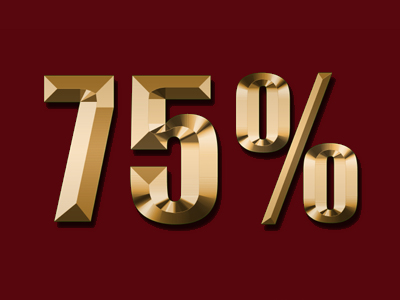75% of 200 is 150.

# Percentages (Year 5)

In Year 5, which is part of KS2 Maths, you'll discover a cool Maths trick! It's all about percentages – turning decimal numbers into percentages. Imagine if halves or quarters were in percentage form – it's like magic!

Numbers have different ways to show their parts – like fractions or decimals. Percentages are like superhero capes for numbers! They tell us how many parts out of 100. Did you know percentages can also give you discounts when you shop? So, learning about percentages is like unlocking secret powers!

How much do you know about percentages? Can you turn decimals and fractions into percentages? Take the super fun quiz to find out!

1.
45% of children in a class are boys. Which percentage were girls?
45%
50%
55%
60%
The total should equal 100%
2.
Which fraction is equivalent to 1%?
120
15
110
1100
Remember that percentage means the number of parts in 100 so 1% = 1100
3.
What is 25% equal to?
12
14
13
15
There are 4 25s in 100 so 25% is the same as 14
4.
Which percentage is 0.75?
25%
30%
70%
75%
0.75 and 75% are both the same as 34
5.
What percentage is one whole?
25%
50%
60%
100%
Percentage means the number of parts in 100 so 100% is 100 parts - that's all of them!
6.
What is 50% equal to?
12
14
13
2
50% is also equal to 0.5
7.
What is 75% of 200?
75
150
225
275
75% of 100 is 75 so 75% of 200 is double 75
8.
How many percent is 0.2?
5%
10%
20%
25%
0.2 and 20% are both the same as 15
9.
What percentage is 110?
10%
20%
25%
100%
10% is also equal to 0.1
10.
Which decimal is equivalent to 25%?
0.1
0.2
0.25
0.5
0.25 and 25% are both the same as 14